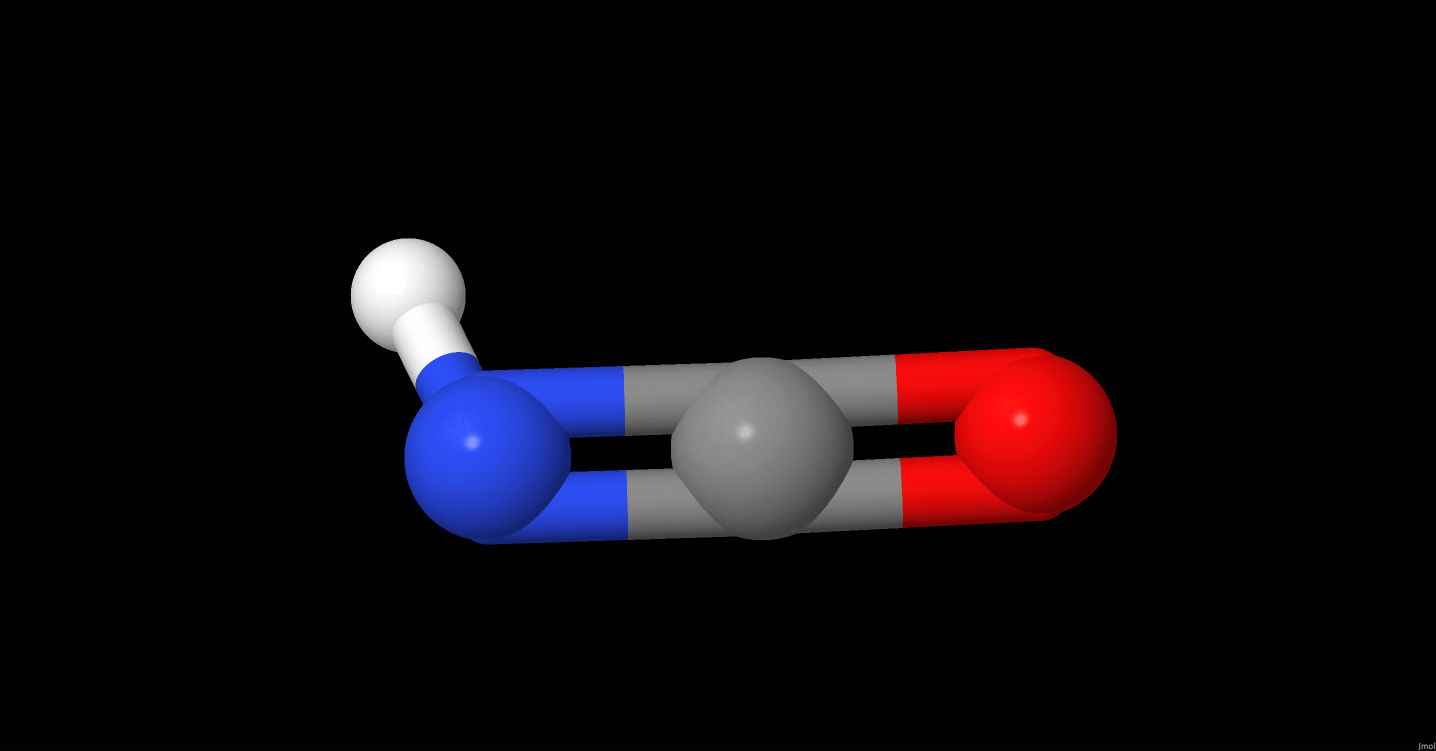# HNCO

Click on the   icon info to get details.

Species data
 Common Formula HNCO Stoichiometric Formula CHNO Name Isocyanic acid Mass 43.00581 a.m.u Charge 0 CAS Inchi InChI=1S/CHNO/c2-1-3/h2H InchiKey OWIKHYCFFJSOEH-UHFFFAOYSA-N State Ground StateISM Abundance
log10 Abundance Reference Source Name Source Type Link
Polarizability
No data
Dipole moment
No data
Enthalpy of formation
 T (K): 0 Value (kJ.mol-1) : -119.05 ±0.37 Method: Reviews and Evaluations Origin: Other database Reference: T (K): 298 Value (kJ.mol-1) : -118.6 ±0.37 Method: Reviews and Evaluations Origin: Other database Reference:
Desorption energy
 Emean (K): 4400 ±1320 E min (K): 0 E max (K): 0 Pre-exponential factor (s-1): 0.00E+0 Method: Calculations Origin: Bibliography Reference: Wakelam, V. et al. ;2017;ArXiv e-prints;, Type of surface: H2O Description: To estimate the unknown binding energies (for most of the radicals for example), we have developed a model founded on the stabilization energy of the complex between the various species and one water molecule. Then, we assume that the binding energy of the species with ASW is proportional to the energy of interaction between this species and one water molecule. To determine the proportionality coefficients, we fit the dependency of the experimental binding energies versus the calculated energies of the complexes for 16 stable molecules. Uncertainties in ED is estimated to be 30%. The preexponential factor is to be computed using the Hasegawa et al. (1992) approximation. Evaluation: Emean (K): 2800 E min (K): 0 E max (K): 0 Pre-exponential factor (s-1): 1.28E+12 Method: Measurements Origin: Bibliography Reference: Quan, D. et al. ;2010;Astrophysical Journal;725,2101-2109 Type of surface: H2O Description: Pre-exponential factor is computed using Hasegawa et al. (1992) Evaluation: Emean (K): 2850 E min (K): 0 E max (K): 0 Pre-exponential factor (s-1): 0.00E+0 Method: Estimation Origin: Other database Reference: Type of surface: H2O Description: This binding energy was listed in the original OSU gas-grain code from Eric Herbst group in 2006. Energy of OCN+H The pre-exponential factor is not given. It can be computed using the formula given in Hasegawa et al. (1992). Evaluation:
Diffusion energy
 E (K): 2946.8 Pre-exponential factor (cm2 s-1): 8.10E-4 Method: Measurements Origin: Bibliography Reference: Mispelaer, F. et al. ;2013;Astronomy & Astrophysics;555, A13 Substrate: H2O ice / Amorphous Type of diffusion: surface Description: These data have been obtained by fitting experimental diffusion rates. The diffusion rates (in cm2s-1) as a function of temperature are listed in Table 3 of Mispelear et al. (2013) and are the following between1.1e-15 and 5e-13 for HNCO (for temperatures between 130 and 140 K). Evaluation: